## ISC Computer Science Previous Year Question Paper 2013 Solved for Class 12

Maximum Marks: 70
Time allowed: 3 hours

Part – I

While answering questions in this Part, indicate briefly your working and reasoning, wherever required.

Question 1.
(a) State the Principle of Duality. Write the dual of: 
(P + Q’).R.1 = P.R + Q’.R
(b) Minimize the expression using Boolean laws: 
F = (A + B’)(B + CD)’
(c) Convert the following cardinal form of expression into its canonical form: 
F (P, Q, R) = π (1, 3)
(d) Using a truth table verify: 
(~p => q) ∧ p = (p ∧ ~q) ∨ (p ∧ q)
(e) If A = 1 and B = 0, then find: 
(i) (A’ + 1).B
(ii) (A + B7
(a) To every Boolean equation there exists another equation which is dual to the previous equation. This is done by changing AND’s to OR’s and vice-versa, 0’s to Fs and vice-versa, complements remain unchanged.
Dual: (P.Q’) + R + 0 = (P + R). (Q’+ R)

(b) F = (A + B’).(B + CD)’
F = (A + B’). (B’. (CD)’)
F = AB’+B’B’.(C’+D’)
F = B’.(C’+D’)

(c) F(P, Q, R) = π(1, 3)
= 001, 011
= (P + Q + R’).(P + Q’ + R’)

(d) (~p => q) ∧ p = (p ∧ ~q) ∨ (p ∧ q)(e) (i) (A’ + 1).B = (0 + 1). 0 = 0
(ii) (A+B’)’ = (1 + 1)’ = (1)’ = 0

Question 2.
(a) Differentiate between throw and throws with respect to exception handling. 
(b) Convert the following infix notation to its postfix form: 
E*(F/(G-H)*I) + J
(c) Write the algorithm for push operation (to add elements) in an array based stack. 
(d) Name the File Stream classes to: 
(i) Write data to a file in binary form.
(ii) Read data from a file in text form.
(e) A square matrix M [ ] [ ] of size 10 is stored in the memory’ with each element requiring 4 bytes of storage. If the base address at M  is 1840, determine the address at M   when the matrix is stored in Row Major Wise. 
(a) Throw: This clause is used to explicitly raise a exception within the program, the statement would throw new exception.
Throws: This clause is used to indicate the exception that are not handled by the method.

(b) E * (F/(G-H) * I) +J
= E*(F/GH- *I) + J
= E * FGH-/I * + J
= EFGH-/I**J +

(c) Step 1: Start
Step 2: if top >= capacity then OVERFLOW, Exit
Step 3: top = top+1
Step 4: Stack [top] = value
Step 5: Stop

(d) (i) FileOutputStream/DataOutputStream/FileWriter/OutputStream
(ii) FileReader / DatalnputStream/ InputStream/ FilelnputStream

M[i] [j] = BA+W [(i – Ir) * column + (j – Ic)]
BA: 1840, Ir = 0, Ic = 0, W = 4, rows = 10, column = 10, i = 4, j = 8
M  = 1840 + 4 [(4 – 0) × 10+ (8 – 0)]
= 1840 + 192
= 2032

Question 3.
(a) The following function Recur is a part of some class. What will be the output of the function Recur () when the value of n is equal to 10. Show the dry run / working. 

void Recur (int n)
{
if (n>1)
{
System.out.print (n + " " );
if(n%2 !=0)
{
n = 3* n + 1;
System.out.print(n + " ");
}
Recur (n/2);
}
}

(b) The following function is a part of some class. Assume ‘n’ is a positive integer. Answer the given questions along with dry run / working,

int unknown (int n)
{
int i, k;
if (n%2 = = 0)
{
i = n/2; k=1;
}
else
{
k=n;
n--;
i=n/2;
}
while (i > 0)
{
k=k*i*n;
i--;
n--;
}
return k;
}

(i) What will be returned by unknown(5)? 
(ii) What will be returned by unknown(6)? 
(iii) What is being computed by unknown (int n)? 
(a) Recur (10)
10 Recur (5)
5
16 Recur (8)
8 Recur (4)
4 Recur (2)
2 Recur (1)
OUTPUT: 10 5 16 8 4 2
(b) (i) 120
(ii) 720
(iii) calculate factorial/ product

Part – II

Answer seven questions in this part, choosing three questions from Section A, two from Section B and two from Section C.

Section – A

Question 4.
(a) Given the Boolean function: F(A, B, C, D) = Σ (0, 2, 4, 5, 8, 9, 10, 12, 13)
(i) Reduce the above expression by using 4-variable K-Map, showing the various groups (i.e. octal, quads and pairs). 
(ii) Draw the logic gate diagram of the reduced expression. Assume that the variables and their complements are available as inputs. [ 1]
(b) Given the Boolean function : F(P, Q, R, S) = Π (0, 1, 3, 5, 7, 8, 9, 10, 11, 14, 15)
(i) Reduce the above expression by using 4-variable K-Map, showing the various groups (i.e. octal, quads and pairs). 
(ii) Draw the logic gate diagram of the reduced expression. Assume that the variables and their complements are available as inputs. 
(a) F(A, B, C, D) = Σ (0, 2, 4, 5, 8, 9, 10, 12, 13)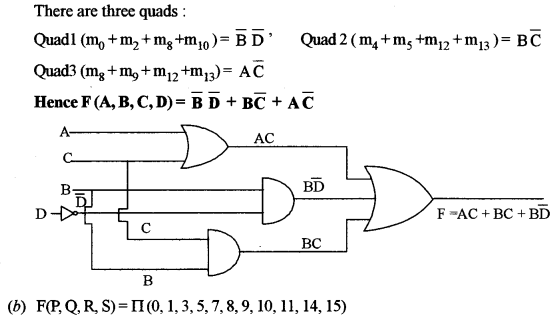Question 5.
A Football Association coach analyzes the criteria for a win/draw of his team depending on the following conditions:
If the Centre and Forward players perform well but Defenders do not perform well.
or
If Goalkeeper and Defenders perform well but the Centre players do not perform well.
or
If all the players perform well.
The inputs are:

 Inputs C Centre players perform well. D Defenders perform well. F Forward players perform well. G Goalkeeper performs well.

(In all of the above cases 1 indicates yes and 0 indicates no)
Output: X – Denotes the win/draw criteria [1 indicates win/draw and 0 indicates defeat in all cases.]
(a) Draw the truth table for the inputs and outputs given above and write the POS expression for X(C, D, F, G). 
(b) Reduce X(C, D, F, G) using Karnaugh’s Map.
Draw the logic gate diagram for the reduced POS expression for X (C, D, F, G ) using AND and OR gate. You may use gates with two or more inputs. Assume that the variable and their complements are available as inputs.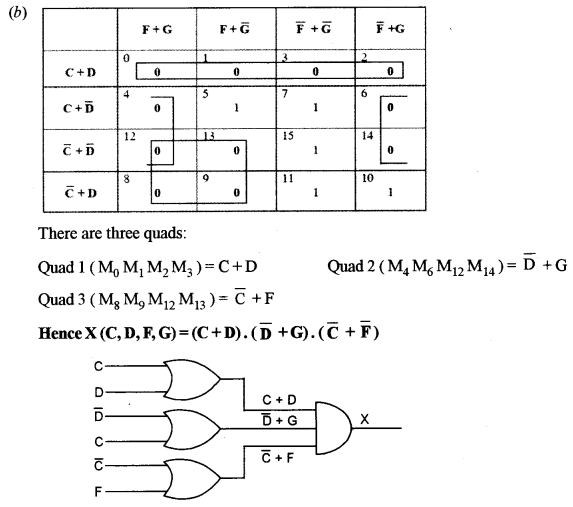Question 6.
(a) In the following truth table, x and y are inputs and B and D are outputs:(i) Write the SOP expression for D.
(ii) Write the POS expression for B.
(iii) Draw a logic diagram for the SOP expression derived for D, using only NAND gates.
(b) Using a truth table, verify if the following proposition is valid or invalid:
(a =>b) ∧ (b =>c) = (a =>c) 
(c) From the logic circuit diagram given below, name the outputs (1), (2) and (3). Finally, derive the Boolean expression and simplify it to show that it represents a logic gate. Name and draw the logic gate.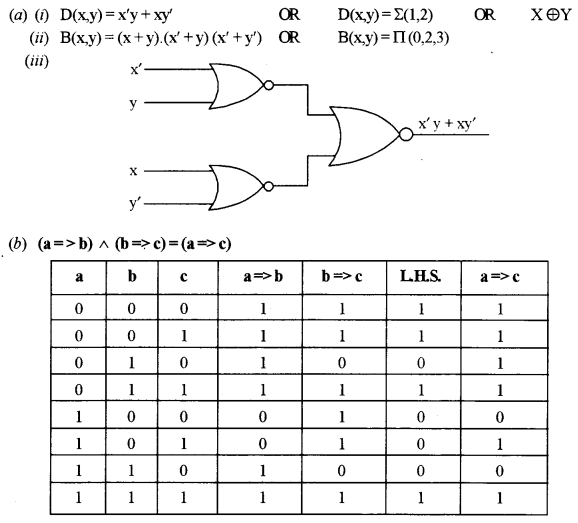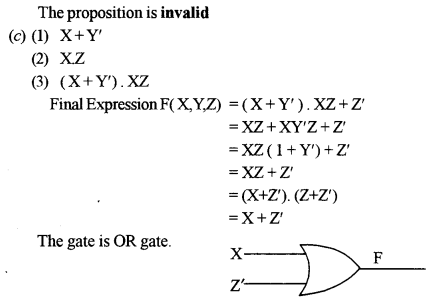Question 7.
(a) What are Decoders? How are they different from Encoders? 
(b) Draw the truth table and a logic gate diagram for a 2 to 4 Decoder and briefly explain its working. 
(c) A combinational logic circuit with three inputs P, Q, R produces output 1 if and only if an odd number of 0’s are inputs. 
(i) Draw its truth table.
(ii) Derive a canonical SOP expression for the above truth table.
(iii) Find the complement of the above-derived expression using De Morgan’s theorem and verify if it is equivalent to its POS expression.
(a) Decoders are a combinational circuit which inputs ‘n’ lines and outputs 2n or fewer lines. Encoders convert HLL to LLL i.e. Octal, Decimal and Hexadecimal to binary whereas Decoders convert LLL to HLL i.e. Binary to Octal, Decimal and Hexadecimal.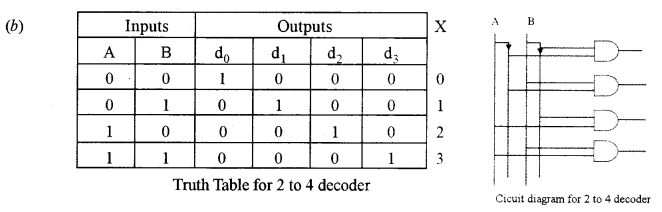Working: If any number is required as output then the inputs should be the binary equivalent. For example, if the input is 01 (A’.B) then the output is 1 and so on.(ii) X (P, Q, R) = P’Q’R’ + P’QR + PQ’R + PQR’
(iii) Complement of X (P, Q, R) = (P + Q + R). (P + Q’ + R’). (P’ + Q + R’). (P’ + Q’ + R) which is not equal to POS expression for the above Truth Table.

Section – B

• Each program should be written in such a way that it clearly depicts the logic of the problem.
• This can be achieved by using mnemonic names and comments in the program.
• Flowcharts and Algorithms are not required
• The programs must be written in Java.

Question 8.
An emirp number is a number which is prime backwards and forwards. Example: 13 and 31 are both prime numbers. Thus, 13 is an emirp number. 
Design a class Emirp to check if a given number is Emirp number or not. Some of the members of the class are given below:
Class name: Emirp
Data members/instance variables:
n: stores the number
rev: stores the reverse of the number
f: stores the divisor
Member functions:
Emirp(int nn): to assign n = nn, rev = 0 and f = 2
int isprime(int x): check if the number is prime using the recursive technique and return 1 if prime otherwise return 0
void isEmirp(): reverse the given number and check if both the original number and the reverse number are prime, by invoking the function isprime(int) and display the result with an appropriate message
Specify the class Emirp giving details of the constructor(int), int isprime (int) and void isEmirp(). Define the main function to create an object and call the methods to check for Emirp number.

import java.util. Scanner;
public class Emirp
{
int n,rev,f;
Emirpfint nn)
{
n=nn;
rev=0;
f=2;
}
intisprime(int x)
{
if(n==x)
{
return 1;
}
else if (n%x = = 0 ||n == 1)
{
return 0;
}
else
return isprime(x+1);
}
void isEmirp()
{
int x=n;
while(x!=0)
{
rev=(rev* 10) + x%10;
x=x/10;
}
int ans1=isprime(f);
n=rev;
f=2;
int ans2=isprime(f);
if(ans 1 ==1 && ans2==1)
System. out.println(n+" is anEmirp number");
else
System.out.println(n+" is not an Emirp number");
}
public static void main()
{
Scanner sc=new Scanner(System.in);
System.out.println("\n Enter a number");
int x=sc.nextInt();
Emirp obj = new Emirp(x);
obj.isEmirp();
}
}

Question 9.
Design a class Exchange to accept a sentence and interchange the first alphabet with the last alphabet for each word in the sentence, with single-letter word remaining unchanged. The words in the input sentence are separated by a single blank space and terminated by a full stop. 
Example:
Input: It is a warm day.
Output: tI si a mraw yad
Some of the data members and member functions are given below:
Class name: Exchange
Data members/instance variables:
sent: stores the sentence
rev: to store the new sentence
size: stores the length of the sentence
Member functions:
Exchange(): default constructor
void readsentence(): to accept the sentence
void exfirstlast(): extract each word and interchange the first and last alphabet of the word and form a new sentence rev using the changed words
void display(): display the original sentence along with the new changed sentence.
Specify the class Exchange giving details of the constructor ( ), void readsentence (), void exfirstlast () and void display (). Define the main () function to create an object and call the functions accordingly to enable the task.

importjava.util.*;
public class Exchange
{
String sent,rev;
int size;
Exchange()
{
sent=null;
rev="";
}
{
Scanner sc=new Scanner(System.in);
System.out.print("\n Enter a sentence ");
sent=sc.nextLine();
size=sent.length();
}
void exfirstlast()
{
int p=0; char ch; String b;
for(inti=0;i<size;i++)
{
ch=sent.charAt(i);
if(ch=="||ch =='.’)
{
b=sent. substring(p,i);
if(b.length() != 1)
{
rev += b.qharAt(b.length()-1);
rev = rev + b.substring(l,b.length()-1);
rev += b.charAt(0);
}
else
rev = rev + b;
rev = rev +" ";
p=i+1;
}
}
}
void display()
{
System.out.print("\n Input: " + sent);
System.out.print("\n Output:" + rev);
}
public static void main()
{
Exchange obj = new Exchange();
obj.exfirstlast();
obj. display();
}
}

Question 10.
A class Matrix contains a two-dimensional integer array of an order [m * n]. The maximum value possible for both ‘m’ and ‘n’ is 25. Design a class Matrix to find the difference between the two matrices. The details of the members of the class are given below: 
Class name: Matrix
Data members/instance variables:
arr[][]: stores the matrix element
m: integer to store the number of rows
n: integer to store the number of columns
Member functions:
Matrix (int mm, int nn): to initialize the size of the matrix m = mm and n = nn
void fillarray(): to enter the elements of the matrix
Matrix SubMat(Matrix A): subtract the current object from the matrix of the parameterized object and return the resulting object
void display(): display the matrix elements
Specify the class Matrix giving details of the constructor(int, int), void fillarray(), Matrix SubMat (Matrix) and void display (). Define the main ( ) function to create objects and call the methods accordingly to enable the task.

import java.util. Scanner;
public class Matrix
{
static Scanner sc=new Scanner(System.in);
int arr[] []=new int ;
int m,n;
Matrix(int mm, int nn)
{
m=mm;
n=nn;
}
voidfillarray()
{
System.out.print("\n Enter elements of array");
for(int i=0;i<m;i++)
{
for(int j=0;j<n; j++)
arr[i] [j]=sc.nextInt();
}
}
Matrix SubMat(Matrix A)
{
Matrix B=new Matrix(m,n);
for(int i=0;i<m;i++)
{
for(int j=0;j<n; j++)
Barr[i][j]= arr[i][j] - A.arr[i][j];
}
return B;
}
void display()
{
for(int i=0;i<m;i++)
{
System.out.println();
{
for(int j=0;j<n;j++)
System, out.print(arr[i][j] +" \t");
}
}
}
public static
void main()
{
System.out.print("\n Size of array");
int x=sc.nextInt();
int y=sc.nextInt();
Matrix A=new Matrix(x, y);
Matrix B=new Matrix(x, y);
Matrix C=new Matrix(x, y);
A.fillarray();
B.fillarray();
C=A.SubMat(B); C.display();
}
}

Section – C

• Answer any two questions Each Program/Algorithm should be written in such a way that it clearly depicts the logic of the problem stepwise. This can also be achieved by using pseudo-codes.
• Flowcharts are not required The programs must be written in Java.
• The Algorithms must be written in general/standard form, wherever required specified

Question 11.
A superclass Perimeter has been defined to calculate the perimeter of a parallelogram. Define a subclass Area to compute the area of the parallelogram by using the required data members of the superclass. The details are given below: 

Class name: Perimeter
Data members/instance variables:
a: to store the length in decimal
b: to store the breadth in decimal
Member functions:
Perimeter (…): parameterized constructor to assign values to data members
double Calculate(): calculate and return the perimeter of a parallelogram is 2* (length + breadth)
void show(): to display the data members along with the perimeter of the parallelogram
Class name: Area
Data members/instance variables:
h: to store the height in decimal
area: to store the area of the parallelogram
Member functions:
Area(…): parameterized constructor to assign values to data members of both the classes
void doarea(): compute the area as (breadth * height)
void show(): display the data members of both classes along with the area and perimeter of the parallelogram.
Specify the class Perimeter giving details of the constructor (…), double Calculate and void show (). Using the concept of inheritance, specify the class Area giving details of the constructor (…), void doarea () and void show (). The main function and algorithm need not be written.

import java.util.*;
class Perimeter
{
protected double a,b;
Perimeter(double aa, double bb)
{
a=aa;
b=bb;
}
double Calculate()
{
return (2*(a+b)); }
void show()
{
System.out.print("\n Length = " + a);
System.out.print("\n Breadth = " + b);
System.out.print("\n Perimeter =" + Calculate());
}
}
importjava.util.*;
class Area extends Perimeter
{
double h;
double area;
Area(double aa, double bb, double cc)
{ super(aa, bb);
h=cc; }
void doarea()
{
area=super.b*h;
}
void show()
{ super, show();
System, out.print("\n Height = " + h);
System.out.print("\n Area = " + area);
}
}

Question 12.
A doubly queue is a linear data structure which enables the user to add and remove integers from either ends, i.e. from front or rear. Define a class Dequeue with the following details: 
Class name: Dequeue
Data members/instance variables:
arr[ ]: array to hold up to 100 integer elements
lim: stores the limit of the dequeue
front: to point to the index of the front end
rear: to point to the index of the rear end
Member functions:
Dequeue(int 1): constructor to initialize the data members lim = 1; front = rear = 0
void addfront(int val): to add integer from the front if possible else display the message (“Overflow from front”) voidaddrear(intval): to add integer from the rear if possible else display the message (“Overflow from rear”)
int popfront(): returns element from front, if possible otherwise returns – 9999
int poprear(): returns element from rear, if possible otherwise returns – 9999
Specify the class Dequeue giving details of the constructor (int), void addfront(int), void addrear (int, popfront ( ) and int poprear ( ). The main function and algorithm need not be written.

public class Dequeue
{
int arr[] = new int;
int lim,front,rear;
Dequeue(int 1)
{
lim=1; front=0; rear=0; arr=newint[lim];
}
{
if(front>0)
arr[front--]=val;
else
System.out.print("\n Overflow from front");
}
{
if(rear<lim-1)
arr[++rear]=val;
else
System.out.print("\n Overflow from rear");
}
int popfront()
{
if(front!=rear)
return arr[++front];
else
return -9999;
}
int poprear()
{
if(front!=rear)
return arr[rear--];
else
return -9999;
}
}

Question 13.
(a) A linked list is formed from the objects of the class: 

class Node
{
int item;
Node next;
}

Write an Algorithm OR a Method to count the number of nodes in the linked list. The method declaration is given below:
int count (Node ptr-start)
(b) What is the Worst Case complexity of the following code segment: 

(i) for(int p = 0;p<N;p++)
{
for (int q=0; q < M; q++)
{
Sequence of statements;
}
}
for(int r=0;r<X;r++)
{
Sequence of statements;
}

(ii) How would the complexity change if all the loops went up to the same limit N?
(c) Answer the following from the diagram of a Binary Tree is given below: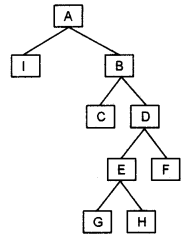(i) Preorder Transversal of the tree. 
(ii) Children of node E. 
(iii) The left subtree of node D. 
(iv) Height of the tree when the root of the tree is at level 0. 
(a) Algorithm to count the number of nodes in a linked list
Steps:
1. Start
2. Set a temporary pointer to the first node and counter to 0.
3. Repeat steps 4 and 5 until the pointer reaches null
4. Increment the counter
5. move the temporary pointer to the next node
6. Return the counter value
7. End
Method to count for the number of nodes in a linked list

int count (Node ptr_start)
{
Node a = new Node(ptr_start);
int c=0;
while (a!=null)
{
c++;
a=a.next;
}
return c:
}

(b) (i) O(N × M) + O(X) OR O(NM + X)
(ii) O( N2) OR O( N2 + N) = O(N2) (by taking the dominant term)
(c) (i) A, I, B, C, D, E, G, H, F
(ii) G and H
(iii) EGH
(iv) 4